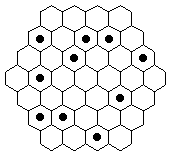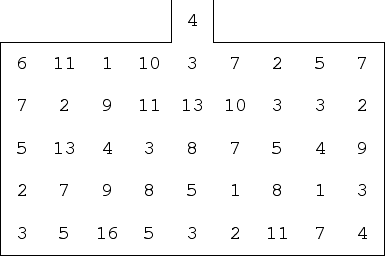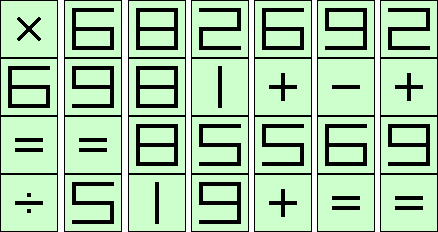2005 U.S. Championship Puzzles

1. Spinners

Divide the hexes into regions each containing exactly one dot, so that each dot is the center of rotational symmetry of its region.2. Factor Maze

Enter the maze from the top. Travel horizontally or vertically, so that each number visited is a factor of the sum of the two preceding numbers. Exit the maze from the top.3. Three Coins

A country mints 3 denominations of coins, in whole numbers of centos. It takes 3 of these coins to make 20 centos, or 23 centos, or 29 centos. What are the denominations of coins?

4. Equation Jigsaw

Cut out and rearrange the 7 vertical strips so that 4 true horizontal equations result.